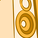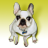# App Development

Discussion board where members can learn more about Qlik Sense App Development and Usage.

Announcements
QlikWorld Online 2021, May 10-12: Our Free, Virtual, Global Event REGISTER TODAY
cancel
Showing results for
Search instead for
Did you mean:Contributor III

## Filter selection issue with Multiple if statement

I have written multiple if statements in two ways to create a master measure.

way 1:

pick(match(Account,'MTDAvgAUM','MTDEndAUM','YTDAvgAUM','YTDEndAUM'),Sum({<Account={'MTDAvgAUM'}, [Period Date]={"\$(=Max([Period Date]))"}, Period=, [Fiscal Month]=, [Fiscal Quarter]=, [Fiscal Year]=>} [Amount])
,Sum({<Account={'MTDEndAUM'}, [Period Date]={"\$(=Max([Period Date]))"}, Period=, [Fiscal Month]=, [Fiscal Quarter]=, [Fiscal Year]=>} [Amount])
,Sum({<Account={'YTDAvgAUM'}, [Period Date]={"\$(=Max([Period Date]))"}, Period=, [Fiscal Month]=, [Fiscal Quarter]=, [Fiscal Year]=>} [Amount])
,Sum({<Account={'YTDEndAUM'}, [Period Date]={"\$(=Max([Period Date]))"}, Period=, [Fiscal Month]=, [Fiscal Quarter]=, [Fiscal Year]=>} [Amount]))

way 2:

Num(
If(Account='MTDAvgAUM', Sum({<Account={'MTDAvgAUM'}, [Period Date]={"\$(=Max([Period Date]))"}, Period=, [Fiscal Month]=, [Fiscal Quarter]=, [Fiscal Year]=>} [Amount])
,If(Account='MTDEndAUM', Sum({<Account={'MTDEndAUM'}, [Period Date]={"\$(=Max([Period Date]))"}, Period=, [Fiscal Month]=, [Fiscal Quarter]=, [Fiscal Year]=>} [Amount])
,If(Account='YTDAvgAUM', Sum({<Account={'YTDAvgAUM'}, [Period Date]={"\$(=Max([Period Date]))"}, Period=, [Fiscal Month]=, [Fiscal Quarter]=, [Fiscal Year]=>} [Amount])
,If(Account='YTDEndAUM', Sum({<Account={'YTDEndAUM'}, [Period Date]={"\$(=Max([Period Date]))"}, Period=, [Fiscal Month]=, [Fiscal Quarter]=, [Fiscal Year]=>} [Amount]),
sum(Amount))))),'#,##0')

But problem is when i am selecting account =MTDAvgAUM in filter , for rest of the if clause it giving 0 which i dont want to show. but i dont want to uncheck include zero value option also.

I want to show only result for selected account.

Please give me some idea how to avoid zero values for rest of the  values.

Thanks

in advance.

Labels (2)

• ### Set Analysis

2 RepliesPartner

Hi,

Not sure if this will work for you but,

You can try using Getcurrentselection() function. See an example below which is working for me:

if(len(GetCurrentSelections(Filter))=0, Amt,

Pick(WildMatch(Filter,'1M', '2M', '3M'),
if(Amt>10 and Amt<=20, Amt,Null()),
if(Amt>20 and Amt<=30, Amt,Null()),
if(Amt>30, Amt,Null())
))

This expression will first check if the selected filter value is 0, so on no selection of filter it will show everything and on selection of filter, it will show only for selected. BUT YOU WILL NEED TO UNCHECK INCLUDE ZERO VALUE option

Check Sheet 3 in the attached file. See the difference by checking and unchecking include zero values.

Regards,

ArjunContributor III
Author

As per my requirement I cant uncheck  include zero value option.

I tried your option but if I select one value, zero or null are coming for rest of the clause which I don't want.

any other way please?